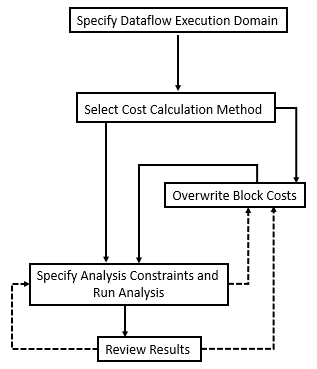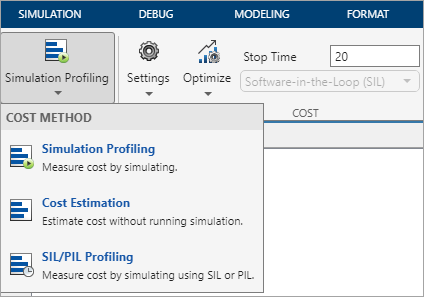## Perform Multicore Analysis for Dataflow

When a subsystem in a model is configured to use a dataflow execution domain, the Multicore tab is activated on the Simulink® toolstrip. This tab consolidates multicore analysis techniques leveraged in dataflow into an incremental and iterative workflow.

Using the controls on the Multicore tab, you can:

• Estimate the relative cost of blocks using internal Simulink heuristics.

• Measure average execution times (cost) of blocks inside the dataflow subsystems by simulating the model with software-in-the-loop (SIL) or processor-in-the-loop (PIL) profiling . This functionality requires an Embedded Coder® license.

• Manually override the block cost values.

• Provide analysis constraints, such as maximum number of threads and threading threshold.

• Run analysis to generate block to threads allocation and visualize analysis results.The chart below illustrates the steps of multicore analysis. After you specify dataflow execution domain for the subsystems in your model, you can select a cost calculation method, overwrite block costs, specify analysis constraints and run analysis, and review results.### Select the Cost Calculation Method

On the Multicore tab, in the Mode section, you can select the method of cost calculation as or . In both modes, the cost of individual blocks will be automatically determined and used in the multicore analysis for equally distributing the computational load across multiple CPU cores.#### Cost Estimation

Use for:

• Quick analysis without running the simulation or generating code.

• Preliminary analysis when the model is not fully implemented. In this case, you can modify the results of the estimation to match the anticipated cost values for the final implementation.

When you click Estimate Cost, the Cost Editor displays the estimated execution cost of each block in your model without simulating it.#### SIL/PIL Profiling

Use the software-in-the-loop (SIL) or processor-in-the-loop (PIL) profiling method (requires Embedded Coder license) to:

• Acquire accurate cost values measured on the host computer using the generated code. The generated code is the closest to the code that will be deployed on the hardware.

• Measure cost values on the actual target hardware in order to maximize the utilization of cores when the final code is deployed.

SIL/PIL profiling measures average execution times (cost) of blocks inside the dataflow subsystems by simulating the model with SIL/PIL.• Use Settings to configure C/C++ code generation and hardware implementation settings.

• Use Stop Time to specify the time to measure the cost.

• Use the drop down menu to select the ```software-in-the-Loop (SIL)``` or` processor-in-the-loop (PIL)` setting.

• Use Profile to measure the costs associated with blocks with the specified settings.

This example shows the highlighted block in the model and its cost.### Manually Change Block Costs

You can manually change the block cost values to understand their impact to the multicore behavior. To override block costs, remove the check in the Auto column for the corresponding block and edit the value in the Cost column.

Overwriting block costs values allows you to perform analysis for custom costs.### Specify Analysis Constraints and Run Analysis

Next, set constraints and run multicore analysis. In the Analyze section:• Use Maximum Number of Threads to specify the maximum number of threads produced by the analysis. By default, the tool tries to automatically determine the number of cores of the target processor from the hardware settings and uses that as maximum number of threads. If the tool is unable to determine the exact value, it will use the number of cores on the host platform as maximum number of threads.

• Specify the Multithreading Threshold to set a minimum for the total cost (in microseconds) of the subsystem, for which the tool applies multithreading. If the total cost falls below the threshold, the tool will not partition the subsystem. By default, the tool uses a nominal value, 25 micro- seconds, as the threshold.

• Click Run Analysis to perform the analysis based on your configuration.

### Review Results

Use the tools provided in the Review Results section to visualize and understand the multicore behavior of your model.Select Highlight threads to highlight and visualize the threads and the assignment of blocks to the threads based on the block execution cost values.#### Use Pipelining to Increase Concurrency

Select Suggestions For Increasing Concurrency to see if there are suggested latencies for pipelining delays. By pipelining the data-dependent blocks, the Dataflow Subsystem block can increase concurrency for higher data throughput. For more information about pipelining delays, see Multicore Simulation and Code Generation of Dataflow Domains.After accepting suggested latencies for pipelining delays, you can use Show pipeline delays to visualize the delays in your model.#### Execution Speedup

Use Execution Speed to indicate the maximum theoretical speedup for the entire model. This speedup can be achieved as a result of the partitioning performed during the analysis.The speedup is calculated using this formula, where `n` is the total number of Dataflow Subsystem blocks, `pctPar` is the percentage of the parallel execution of a subsystem, and `criticalPathCost` is the cost of the most costly thread in a subsystem.

`$Speedup\le \frac{1}{\left(1-\sum _{i=0}^{n}pctPa{r}_{i}\right)+\sum _{i=0}^{n}\frac{pctPa{r}_{i}×criticalPathCos{t}_{i}}{totalCostInSubsyste{m}_{i}}}$`